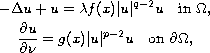Electron. J. Diff. Eqns., Vol. 2006(2006), No. 131, pp. 1-15.

### A semilinear elliptic problem involving nonlinear boundary condition and sign-changing potential Tsung-fang Wu

Abstract:
In this paper, we study the multiplicity of nontrivial nonnegative solutions for a semilinear elliptic equation involving nonlinear boundary condition and sign-changing potential. With the help of the Nehari manifold, we prove that the semilinear elliptic equation:has at least two nontrivial nonnegative solutions foris sufficiently small.

Submitted July 6, 2006. Published October 17, 2006.
Math Subject Classifications: 35J65, 35J50, 35J55.
Key Words: Semilinear elliptic equations; Nehari manifold; Nonlinear boundary condition.

Show me the PDF file (255K), TEX file, and other files for this article.Tsung-Fang Wu Department of Applied Mathematics National University of Kaohsiung Kaohsiung 811, Taiwan email: tfwu@nuk.edu.tw